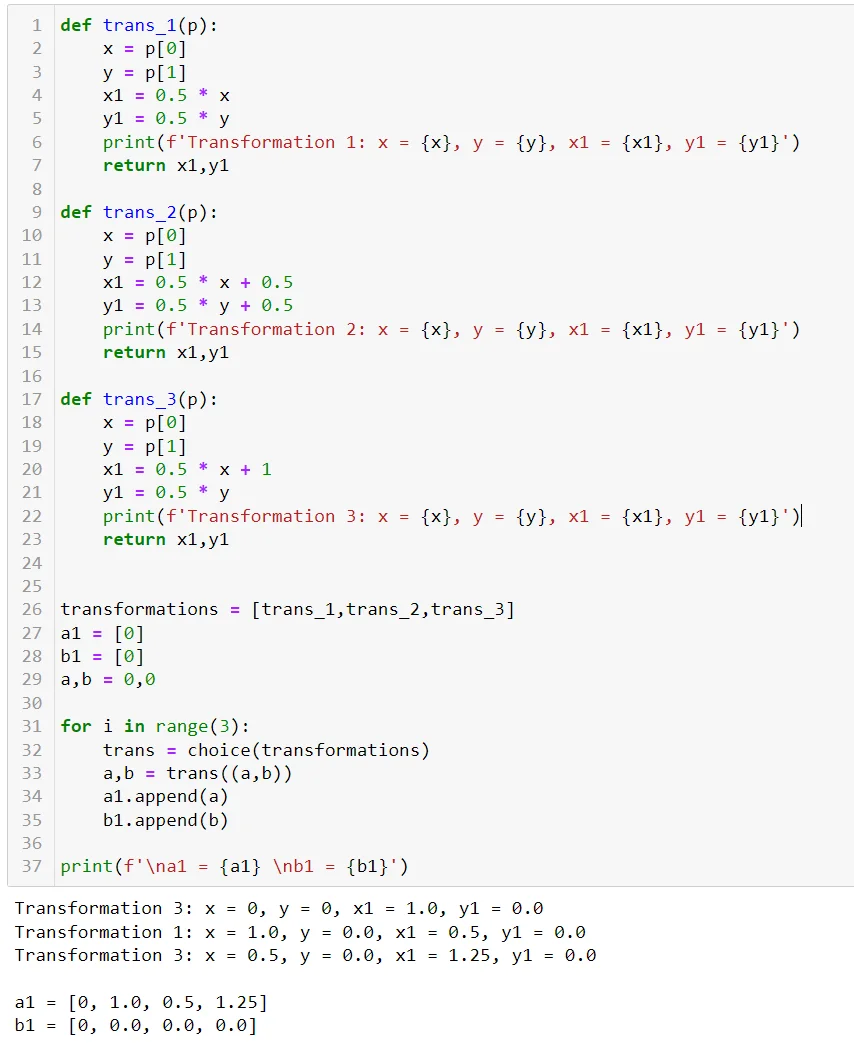05 Aug 2022

Posted on:

03 Aug 2022

0

# Resolved:Questions about the transformation function

On the line:

``````a,b = trans((a,b))
``````

I understand that the p becomes (a,b), but what happens next?
Ler's say we take `trans_3`.  Can someone explains what happens inside the function when we call `trans_3((a,b))`?

Instructor
Posted on:

04 Aug 2022

2

Hey Bondan,

Let me suggest a modification to the code, where I have included 3 `print`-statements on lines 6, 14, 22, and 37.Then, let's study the `for`-loop on lines 31-35, where I have reduced the number of iterations to 3.
On line 32, we assign the variable `trans` one of the 3 functions - `trans_1`, `trans_2`, or `trans_3`. From the output, we can see that the choice during the first iteration was `trans_3`. On line 33, we modify the integers `a` and `b` using the function `trans_3`. What happens in this function is the following:
1. The variable `p` represents the tuple `(a, b)`
2. Line 18 - `x` equals the first item in `p`, namely `a`
3. Line 19 - `y` equals the second item in `p`, namely `b`
4. Line 20 - `x1` is computed according to Transformation 3, using variable `x`
5. Line 21 - `y1` is computed according to Transformation 3, using variable `y`
6. Line 23 - the function returns the variables `x1` and `y1` in a tuple
Going back to line 33, variable `a` is assigned the value `x1` while variable `b` is assigned the value `y1`. These are then appended to the lists `a1` and `b1`, respectively.
Then, the second iteration comes, where, this time, the function `trans_1` is chosen. The whole procedure from above repeats.
Finally, `trans_3` is chosen again during the third iteration.

Hope this helps!

Kind regards,
365 Hristina

Posted on:

05 Aug 2022

0

Oh I get it now! It's like calling multiple items wtih one variable. Thank you for the answer Hristina